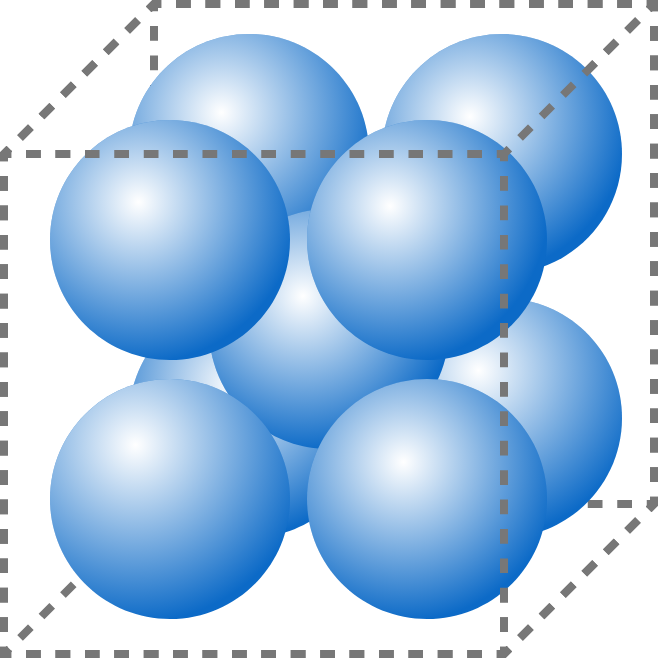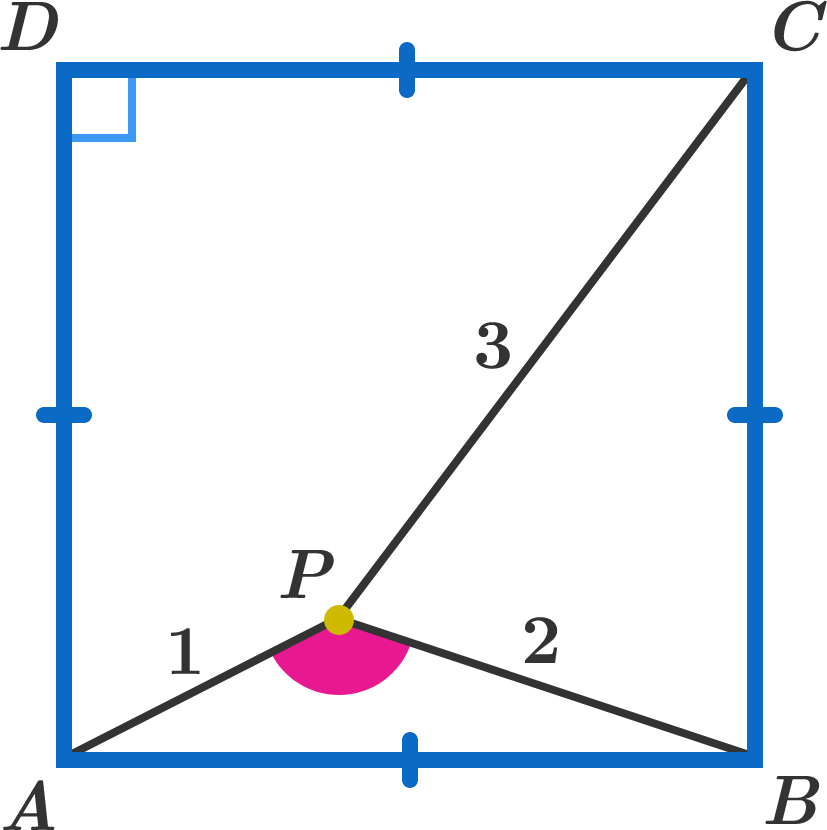# Problems of the Week

Contribute a problem

# 2017-09-04 Intermediate

Are there distinct positive integers $m$ and $n$ such that $mn$ evenly divides $m^2+n^2?$9 identical, spherical balls are packed into a cube of edge length 100.

The 8 balls in corners are each tangent to the three faces making up its corner, and the $9^\text{th}$ ball is tangent to the other 8.

What is the radius of the spherical balls, to 1 decimal place?

Let $n$ be an integer randomly chosen in the interval $[1, 120]$, and consider the sum $S_n = \displaystyle \sum_{k=1}^n k.$

$S_n$ is least likely to be divisible by $\text{\_\_\_\_\_\_\_\_\_\_}.$

Point $P$ is inside square $ABCD$ such that $AP=1, BP=2, CP=3.$

Find $\angle APB$ in degrees.A student randomly chooses $m$ distinct numbers from among the first 2017 positive integers and writes them all on a chalkboard.

She then chooses two of the numbers written on the chalkboard, erases them both, and writes down their least common multiple. She repeats this process until only one number remains on the chalkboard.

What is the smallest integer $m$ such that the final number is guaranteed to be a multiple of 128?

It may be helpful to note that $128=2^{7}$ and 2017 is prime.An example of the process with $m=4.$

Bonus: Can you come up with a simple formula for the smallest $m$ such that the final number is a multiple of $n$, where $n$ is a positive integer?

×